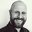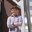Question-and-Answer Resource for the Building Energy Modeling Community
Get started with the Help page

# When would it make sense to use a non-default surface convection algorithm?There are four SurfaceConvectionAlgorithm:Inside and five SurfaceConvectionAlgorithm:Outside options for calculating surface convection in EnergyPlus. The default for inside is TARP and for outside is DOE-2 and I've never really considered trying the other options.

My question is what are the differences between the algorithms (in theory and in practice)?

• Do some run faster than others?

• Are some better-validated than others?

• Do some capture different effects?

• Are some more robust to errors/inaccuracies in input parameters?

• How large are the differences (in energy use or indoor conditions) when using different algorithms?

Essentially, under what situations would it make sense to use anything other than the default options?

edit retag close merge delete

Sort by » oldest newest most votedThis is only a partial answer, but as far a speed goes, any algorithm that doesn't involve a $\Delta T$ term will allow E+ to run faster on average. The models that include a $\Delta T$ term are accounting for buoyancy driven natural convection. For a given surface, the convection contribution to the energy balance equation is $Q_c = h_c \Delta T$. This is a linear expression only when $h_c$ doesn't depend on temperature, and linear equations can be solved with one iteration. Also equations that are near-linear can be solved faster than highly nonlinear ones. So a model with $h_c \sim \sqrt { \Delta T }$ will generally run faster than one with $h_c \sim \ { \Delta T } ^2$. This also relates to the robustness because a more linear model is more likely to help the heat balance convergence.

more